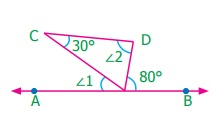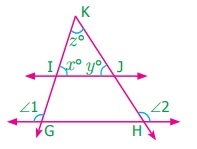Home | | Maths 7th Std | Exercise 5.6

# Exercise 5.6

7th Maths : Term 1 Unit 5 : Geometry : Exercise 5.6 : Miscellaneous Practice problems, Challenge Problems, Text Book Back Exercises Questions with Answers, Solution

Exercise 5.6

Miscellaneous Practice problems

1. Find the value of x if AOB is a right angle.AOC + COB = 90°

2x + 3x = 90°

5x = 90°

x = 90° / 5 = 18°

x = 18°

2. In the given figure, find the value of x.BOC + AOC = 180°

2x + 23 + 3x – 48 = 180°

5x – 25 = 180°

5x = 180° + 25o

= 205o

x = 205o / 5 = 41°

x = 41°

3. Find the value of x, y and zBOD + BOC = 180°

x + 3x + 48 = 180°

4x + 40 = 180°

4x = 180° – 40o

= 140o

x = 140o / 4 = 35°

x = 35°

BOD + AOD = 180°

x + y + 30° = 180°

35° + y + 30° = 180°

y = 180° – 65o

y = 115o

AOD + AOC = 180°

y + 30° + z + 10° = 180°

115° + z + 40° = 180°

z + 155° = 180°

z = 180° – 155° = 25°

z = 25°

4. Two angles are in the ratio 11: 25. If they are linear pair, find the angles.

Two angles are in the ratio 11:25

Let the two angles be 11x, 25x

Sum of two angles 11x + 25x = 180°

36x = 180°

x = 180o / 36 = 5°

x = 5°

11x = 11 × 5° = 55°

25x = 25 × 5° = 125°

The two angles are 55o, 125o(i) Is 1 adjacent to 2?

(ii) Is AOB adjacent to BOE?

(iii) Does BOC and BOD form a linear pair?

(iv) Are the angles COD and BOD supplementary?

(v) Is 3 vertically opposite to 1?

(i) Is l adjacent to 2?

Yes. They have a common vertex, a common arm and their interiors do not overlap.

(ii) Is AOB adjacent to BOE?

No. They have overlapping interiors.

(iii) Do BOC and BOD form a linear pair?

No. Since BOC is a straight angle, their sum will exceed 180°.

(iv) Are COD and BOD supplementary?

Yes. They are linear pair.

(v) Is 3 vertically opposite to 1 ?

No. They are not formed by intersecting lines.

6. In the figure POQ, ROS and TOU are straight lines. Find the x°.UOV + VOP + POR + ROT = 180°

x + 45° + 47° + 36° = 180°

x + 128° = 180°

x = 180° – 128° = 52°

x = 52o

7. In the figure AB is parallel to DC. Find the value of 1 and 2. Justify your answer.From the figure, DCE = 1 (alternate angles)

30° = l

l = 30°

2 = DEB (alternate angles)

2 = 80°

8. In the figure AB is parallel to CD. Find x,y and z.From the figure,

GED = CEF (vertically opposite angles)

y = 42°

CEF = EFB (alternate angles)

42° = z

z = 42°

AFC + CFE + EFB = 180°

x° + 63°+ z° = 180°

x° + 63° + 42° = 180°

x° + 105° =180°

x° = 180°– 105° = 75°

x° = 75°

x° = 75°, y = 42°, z = 42°

9. Draw two parallel lines and a transversal. Mark two alternate interior angles G and H. If they are supplementary, what is the measure of each angle?

Angles G and H are supplementary

G and H are alternate interior angles.

G = H = 90°

10. A plumber must install pipe 2 parallel to pipe 1. He knows that 1 is 53. What is the measure of 2?1 + 2 = 180°

53° + 2 = 180°

2 = 180° – 53° = 127°

2 = 127°

Challenge Problems

11. Find the value of y.POT + TOS + SOR + ROQ = 180o

60° + 3y – 20 + y + y + 10 = 180°

60° + 10 – 20 + 5y = 180°

70 – 20 + 5y = 180°

50 + 5y = 180°

5y = 180° – 50° = 130°

y = 130° / 5 = 26o

y = 26°

12. Find the value of z.POQ + QOM + MON + PON = 360o

3z + 4z –25 + z + 10 +2z – 5 = 360o

3z + 4z + z + 2z + 10 – 25 – 5 = 360o

10z – 20 = 360o

10z = 360o + 20o = 380o

z = 380o / 10 = 38o

z = 38o

13. Find the value of x and y if RS is parallel to PQ.From the figure,

POR = ORS (alternate angles)

POR = 25°

POR = QOU (vertically opposite angles)

QOU = 25°

y = 25°

POT = SOQ (vertically opposite angles)

40° = SOQ

RSO = SOQ (alternate angles)

x° = 40°

y° = 25°

14. Two parallel lines are cut by a transversal. For each pair of interior angles on the same side of the transversal, if one angle exceeds the twice of the other angle by 48°. Find the angles.

Given one angle exceeds twice of other angle by 48°

= 2x + 48

Other angle = x°

Sum of interior angles = x° + 2x° + 48° = 180°

3x + 48° = 180°

3x = 180° – 48° = 132°

x = 132o / 3 = 44o

x = 44°

2x + 48 = 2 × 44 + 48

= 88 + 48° = 136°

The angles are 44°, 136°.

15. In the figure, the lines GH and IJ are parallel. If 1=108° and 2 = 123°, find the value of x, y and z.1 = 108°

Interior angle          G = 180o – 108o = 72o

x° = Interior angle   G (corresponding angles)

x° = 72°

2 = 123°

Interior angle               H = 180° – 123° = 57°

y° = Interior angle       H = (corresponding angles)

y = 57°

x° + y° + 2° = 180° (sum of the triangles)

72° + 57° + z° = 180°

129° + z° = 180°

z° = 180° – 129° = 51°

x° = 72°, y° = 57°, z = 51°

16. In the parking lot shown, the lines that mark the width of each space are parallel. If 1 = (2x–3y)°, 2 = (x +39)°, find x and y.2 + 65° = 180° (interior angles)

(x + 39) + 65° = 180°

x + 104° = 180°

x = 180° – 104° = 76°

x = 76°

1= 65° (vertically opposite angles)

(2x – 3y)o = 65°

2(76o) – 3yo = 65°

152o – 3yo = 65o

–3y° = 65° – 152° = –87o

y = –87 / –3 = 29°

y = 29°

x = 76° , y = 29°

17. Draw two parallel lines and a transversal. Mark two corresponding angles A and B. If A = 4x and B = 3x + 7, find the value of x. Explain..

A and B are corresponding angles.

A = B

4x = 3x + 7

4x – 3x = 7

x = 7°

18. In the figure AB is parallel to CD. Find x˚, y˚ and z˚.A = D (alternate angles)

x° = 48°

C = B (alternate angles)

y° = 60°

from ΔCDE,

C + D + E = 180° (sum of the three angles of a triangle)

60° + 48° + E = 180°

108° + E = 180°

E = 180° – 108°

E = 72°

E + Z° = 180° (straight angles)

72° + Z° = 180°

Z° = 180° – 72° = 108°

Z° =108°

x = 48°     y = 60°    z = 108°

19. Two parallel lines are cut by a transversal. If one angle of a pair of corresponding angles can be represented by 42˚ less than three times the other. Find the corresponding angles.

One angle = x

Other angle = 42 less than 3 times the other

= 3x – 42

The corresponding angles are equal

3x – 42 = x

3x – x = 42

2x = 42

x = 21

The corresponding angles = 21

20. In the given figure, 8 = 107˚, what is the sum of the 2 and 4?8 = 4 (Corresponding angles)

8 = 107°

4 = 107°

4 = 2 (vertically opposite angles)

2 = 107°

The sum of 2 and 4 = 107° + 107° = 214°.

Exercise 5.6

1. 18°

2. 41°

3. 35°, 115°, 25°

4. 55°, 125°

5. (i) Yes. They have a common vertex, a common arm and their interiors do not overlap.

(ii) No. They have overlapping interiors.

(iii) No. Since BOC is a straight angle, their sum will exceed 180°.

(iv) Yes. They are linear pair.

(v) No. They are not formed by intersecting lines

6. 52°

7. 30°, 80°

8. 75° , 42° , 42°

9. 90°

10. 127°

Challenge Problems

11. 26°

12. 38°

13. 40°,25°

14. 44°

15. 72°,57°,51°

16. 76°,29°

17. 7°

18. 48°,60°,108°

19. 21°

20. 214°

Tags : Questions with Answers, Solution | Geometry | Term 1 Chapter 5 | 7th Maths , 7th Maths : Term 1 Unit 5 : Geometry
Study Material, Lecturing Notes, Assignment, Reference, Wiki description explanation, brief detail
7th Maths : Term 1 Unit 5 : Geometry : Exercise 5.6 | Questions with Answers, Solution | Geometry | Term 1 Chapter 5 | 7th Maths